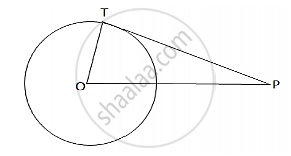# A Point P is 25 Cm Away from the Center of a Circle and the Length of Tangent Drawn from P to the Circle is 24 Cm. Find the Radius of the Circle. - Mathematics

A point P is 25 cm away from the center of a circle and the length of tangent drawn from P to the circle is 24 cm. Find the radius of the circle.

#### SolutionDraw a circle and let P be a point such that OP = 25cm.
Let TP be the tangent, so that TP = 24cm
Join OT where OT is radius.

Now, tangent drawn from an external point is perpendicular to the radius at the point of contact.
∴  OT ⊥ PT
In the right Δ OTP,we have:

OP^2 = OT^2 +TP^2    [By Pythagoras’ theorem:]

OT^2 =  sqrt(OP^2 - TP^2 )

=sqrt(25^2 - 24^2

= sqrt(625-576)

=sqrt(49)

= 7 cm

∴ The length of the radius is 7cm.

Concept: Concept of Circle - Centre, Radius, Diameter, Arc, Sector, Chord, Segment, Semicircle, Circumference, Interior and Exterior, Concentric Circles
Is there an error in this question or solution?